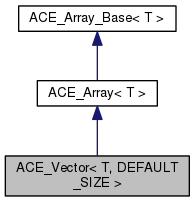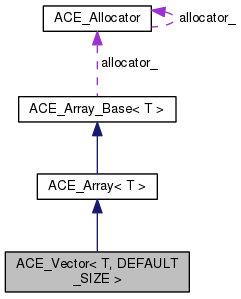ACE  6.2.1
ACE_Vector< T, DEFAULT_SIZE > Class Template Reference

Defines an STL-like vector container. More...

`#include <Vector_T.h>`

Inheritance diagram for ACE_Vector< T, DEFAULT_SIZE >:[legend]
Collaboration diagram for ACE_Vector< T, DEFAULT_SIZE >:[legend]

## Public Types

typedef ACE_Vector_Iterator< T,
DEFAULT_SIZE >
IteratorPublic Types inherited from ACE_Array< T >
typedef T TYPE

typedef ACE_Array_Iterator< T > ITERATORPublic Types inherited from ACE_Array_Base< T >
typedef T TYPE

typedef ACE_Array_Iterator< T > ITERATOR

typedef T value_type

typedef value_typeiterator

typedef value_type const * const_iterator

typedef value_typereference

typedef value_type const & const_reference

typedef value_typepointer

typedef value_type const * const_pointer

typedef ptrdiff_t difference_type

typedef ACE_Allocator::size_type size_type

## Public Member Functions

ACE_Vector (const size_t init_size=DEFAULT_SIZE, ACE_Allocator *alloc=0)

~ACE_Vector ()

size_t capacity (void) const

size_t size (void) const

void clear (void)

void resize (const size_t new_size, const T &t)

void push_back (const T &elem)

void pop_back (void)

void dump (void) const

bool operator== (const ACE_Vector< T, DEFAULT_SIZE > &s) const
Equality comparison operator. More...

bool operator!= (const ACE_Vector< T, DEFAULT_SIZE > &s) const
Inequality comparison operator. More...

void swap (ACE_Vector &rhs)Public Member Functions inherited from ACE_Array< T >
ACE_Array (size_t size=0, ACE_Allocator *alloc=0)
Dynamically create an uninitialized array. More...

ACE_Array (size_t size, const T &default_value, ACE_Allocator *alloc=0)
Dynamically initialize the entire array to the {default_value}. More...

ACE_Array (const ACE_Array< T > &s)
Copy constructor. More...

void operator= (const ACE_Array< T > &s)
Assignment operator. More...

bool operator== (const ACE_Array< T > &s) const
Equality comparison operator. More...

bool operator!= (const ACE_Array< T > &s) const
Inequality comparison operator. More...Public Member Functions inherited from ACE_Array_Base< T >
ACE_DECLARE_STL_REVERSE_ITERATORS ACE_Array_Base (size_type size=0, ACE_Allocator *the_allocator=0)
Dynamically create an uninitialized array. More...

ACE_Array_Base (size_type size, T const &default_value, ACE_Allocator *the_allocator=0)
Dynamically initialize the entire array to the default_value. More...

ACE_Array_Base (ACE_Array_Base< T > const &s)

void operator= (ACE_Array_Base< T > const &s)

~ACE_Array_Base (void)
Clean up the array (e.g., delete dynamically allocated memory). More...

T & operator[] (size_type slot)

T const & operator[] (size_type slot) const

int set (T const &new_item, size_type slot)

int get (T &item, size_type slot) const

size_type size (void) const
Returns the <cur_size_> of the array. More...

int size (size_type new_size)

size_type max_size (void) const
Returns the <max_size_> of the array. More...

int max_size (size_type new_size)

void swap (ACE_Array_Base< T > &array)

iterator begin (void)

iterator end (void)

const_iterator begin (void) const

const_iterator end (void) const

reverse_iterator rbegin (void)

reverse_iterator rend (void)

const_reverse_iterator rbegin (void) const

const_reverse_iterator rend (void) const

## Protected Attributes

size_t length_

size_t curr_max_size_

## Friends

class ACE_Vector_Iterator< T, DEFAULT_SIZE >Protected Member Functions inherited from ACE_Array_Base< T >
bool in_range (size_type slot) const

## Detailed Description

### template<class T, size_t DEFAULT_SIZE = ACE_VECTOR_DEFAULT_SIZE> class ACE_Vector< T, DEFAULT_SIZE >

Defines an STL-like vector container.

This is an STL-like template vector container, a wrapper around ACE_Array. It provides at least the basic std::vector look and feel: push_back(), clear(), resize(), capacity(). This template class uses the copy semantic paradigm, though it is okay to use reference counted smart pointers (see ACE_Ptr<T>) with this template class.

Requirements and Performance Characteristics

• Internal Structure ACE_Array
• Duplicates allowed? Yes
• Random access allowed? No
• Search speed N/A
• Insert/replace speed Linear
• Iterator still valid after change to container? Yes
• Frees memory for removed elements? No
• Items inserted by Value
• Requirements for contained type
1. Default constructor
2. Copy constructor
3. operator=

## Member Typedef Documentation

template<class T , size_t DEFAULT_SIZE = ACE_VECTOR_DEFAULT_SIZE>
 typedef ACE_Vector_Iterator ACE_Vector< T, DEFAULT_SIZE >::Iterator

A short name for iterator for ACE_Vector.

## Constructor & Destructor Documentation

template<class T , size_t DEFAULT_SIZE>
 ACE_Vector< T, DEFAULT_SIZE >::ACE_Vector ( const size_t init_size = `DEFAULT_SIZE`, ACE_Allocator * alloc = `0` )
inline

General constructor.

Parameters
 init_size Initial size of the vector with the default value of DEFAULT_SIZE alloc Pointer to an ACE allocator. If it is NULL then the default ACE allocator is used
template<class T , size_t DEFAULT_SIZE>
 ACE_Vector< T, DEFAULT_SIZE >::~ACE_Vector ( )
inline

Destructor.

## Member Function Documentation

template<class T , size_t DEFAULT_SIZE>
 size_t ACE_Vector< T, DEFAULT_SIZE >::capacity ( void ) const
inline

Returns the current vector capacity, that is, the currently allocated buffer size.

Returns
Current buffer size of the vector
template<class T , size_t DEFAULT_SIZE>
 void ACE_Vector< T, DEFAULT_SIZE >::clear ( void )
inline

Clears out the vector. It does not reallocate the vector's buffer, it is just sets the vector's dynamic size to 0.

template<class T , size_t DEFAULT_SIZE>
 void ACE_Vector< T, DEFAULT_SIZE >::dump ( void ) const

This function dumps the content of the vector. TO BE MOVED out of this class. It needs to be implemented as a global template function that accepts a const ACE_Vector<T>, in order to make instances of this class compile on Linux, AIX. G++ and xlC have template instantiation algoriths, which are different from the one in Visual C++. The algorithms try to instantiate ALL methods declared in the template class, regardless of whether the functions are used or not. That is, all of the classes, that are used as elements in ACE_Vector's, have to have the dump() methods defined in them (seems to be overkill).

This function calls T::dump() for each element of the vector.

template<class T , size_t DEFAULT_SIZE>
 bool ACE_Vector< T, DEFAULT_SIZE >::operator!= ( const ACE_Vector< T, DEFAULT_SIZE > & s ) const
inline

Inequality comparison operator.

Compare this vector with

• s for inequality such that `*this` !=
• s is always the complement of the boolean return value of `*this` ==
• s.
template<class T , size_t DEFAULT_SIZE>
 bool ACE_Vector< T, DEFAULT_SIZE >::operator== ( const ACE_Vector< T, DEFAULT_SIZE > & s ) const

Equality comparison operator.

Compare this vector with

• s for equality. Two vectors are equal if their sizes are equal and all the elements are equal.
template<class T , size_t DEFAULT_SIZE>
 void ACE_Vector< T, DEFAULT_SIZE >::pop_back ( void )
inline

Deletes the last element from the vector ("pop back"). What this function really does is decrement the dynamic size of the vector. The vector's buffer does not get reallocated for performance.

template<class T , size_t DEFAULT_SIZE>
 void ACE_Vector< T, DEFAULT_SIZE >::push_back ( const T & elem )

Appends a new element to the vector ("push back"). If the dynamic size of the vector is equal to the capacity of the vector (vector is at capacity), the vector automatically doubles its capacity.

Parameters
 elem A reference to the new element to be appended. By default, this parameters gets initialized with the default value of the class T.
template<class T , size_t DEFAULT_SIZE>
 void ACE_Vector< T, DEFAULT_SIZE >::resize ( const size_t new_size, const T & t )

Resizes the vector to the new capacity. If the vector's current capacity is smaller than the size to be specified, then the buffer gets reallocated. If the new capacity is less than the current capacity of the vector, the buffer size stays the same.

Parameters
 new_size New capacity of the vector t A filler value (of the class T) for initializing the elements of the vector with. By default, if this parameter is not specified, the default value of the class T will be used (for more detail, see the initialization clause for this parameter).
template<class T , size_t DEFAULT_SIZE>
 size_t ACE_Vector< T, DEFAULT_SIZE >::size ( void ) const
inline

Returns the vector's dynamic size / actual current size of the vector. Do not confuse it with ACE_Array::size(), which returns the array's capacity. Unfortunately, ACE is not very consistent with the function names.

Returns
Dynamic size / actual current size of the vector.
template<class T , size_t DEFAULT_SIZE>
 void ACE_Vector< T, DEFAULT_SIZE >::swap ( ACE_Vector< T, DEFAULT_SIZE > & rhs )
inline

## Friends And Related Function Documentation

template<class T , size_t DEFAULT_SIZE = ACE_VECTOR_DEFAULT_SIZE>
 friend class ACE_Vector_Iterator< T, DEFAULT_SIZE >
friend

## Member Data Documentation

template<class T , size_t DEFAULT_SIZE = ACE_VECTOR_DEFAULT_SIZE>
 size_t ACE_Vector< T, DEFAULT_SIZE >::curr_max_size_
protected

Current capacity (buffer size) of the vector.

template<class T , size_t DEFAULT_SIZE = ACE_VECTOR_DEFAULT_SIZE>
 size_t ACE_Vector< T, DEFAULT_SIZE >::length_
protected

Dynamic size (length) of the vector.

The documentation for this class was generated from the following files: